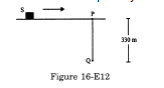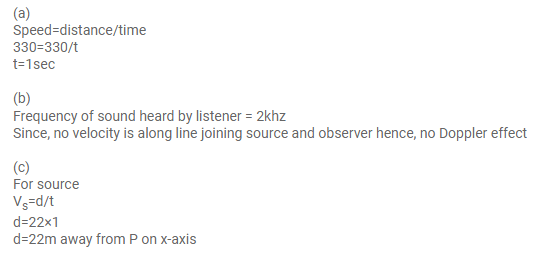# Figure shows a source of sound moving alongQuestion:

Figure shows a source of sound moving along $X$-axis at a speed of $22 \mathrm{~m} \mathrm{~s}^{-1}$ continuously emitting a sound of frequency $2.0$ $\mathrm{kHz}$ which travels in air at a speed of $330 \mathrm{~m} \mathrm{~s}^{-1}$. A listener $Q$ stands on the $Y$-axis at a distance of $330 \mathrm{~m}$ from the origin. At $t$ $=0$, the sources crosses the origin $P$. (a) When does the sound emitted from the source at $P$ reach the listener $Q$ ? (b) What will be the frequency heard by the listener at this instant? (c) Where will the source be at this instant?Solution: